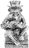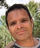### Inconsitancy by data aggregation

Hello,

Ninox newbie here so please be gentle :) I have imported a lot of a data and want to clean out data inconsistancies.  This case is fairly simple, for each invoice the invoice amount must matche the sum of its invoice items amount plus the invoice delivery costs.

The problem I am having is that "if" in the statement below, for some invoices it displays differences, but when I print these out the values are the same? I have tried comparing these as numbers or text, as many decimals as possible, you name it ... and I don't have consistant results, its driving me crazy.

The following is the code which I run from the console:

for i in (select Invoices['Auction House'.name = "eBay"] order by 'Purchase Date') do
let total := sum((select 'Invoice Items'['Invoices' = i]).'Invoice Line Amount IC')

let c := number(total + i.'Delivery Costs IC')
if number(i.'Invoice Amount IC') != number(c) then
format(number(i.'Invoice Amount IC'),"0.0000000") + " != " + format(number(c),"0.000000")
end
end

Which outputs the following (incorrect) lines:

40.9400000 != 40.940000
88.9800000 != 88.980000

Can anyone explain this behaviour?

Thanks,

IldeThere is a known issue with number precision...

https://ninox.com/en/forum/technical-help-5ab8fe445fe2b42b7dd39ee7/managing-precision-in-number-field-seeing-non-exact-values.-5dbeed16466081700bd68940

If you increased the precision you might see it. Do you need more than 2 decimal places?It looks like Ninox isn't the only one with this issue...Well, it looks like I stepped on it again. First, I took more time to read the Google doc that I posted a link for and they do have a data type that can store numbers correctly, NUMERIC. I focused on the FLOAT64 data type and that matched what was going on in the threads I posted the link for. Second, I went back to the old Ninox threads and tried to duplicate the issues and couldn't. It appears that they have resolved number storage problem.

Ilde, I'm not sure why you are using the number() function when you are already dealing with numbers or have already converted to a number. I also don't know if it makes any difference.

Hi Sean, thank you for reply.  Yes indeed the number() and format() are unncesary, I was trying to determine where the issue could be.  I only need 2 digits, but I expended to 5 to see if the precision was actually the problem.  The fields are defined as numeric in the table, the code on its bear minimum should be:

for i in (select Invoices['Auction House'.name = "eBay"] order by 'Purchase Date') do
let total := sum((select 'Invoice Items'['Invoices' = i]).'Invoice Line Amount IC')

let t1 := i.'Invoice Amount IC'
let t2 := total + i.'Delivery Costs IC'

if t1 != t2 then
t1 + " != " + t2
end
end

Which yields the following (incorrect) result:

60.16 != 62.16
173.98 != 187.97
135.98 != 135.98
22.95 != 27.95
71.35 != 78.35
40.99 != 40.99
45.97 != 50.97
32.95 != 30.00
40.94 != 40.94
88.98 != 88.98

Next I tried with the following:

let t1 := text(i.'Invoice Amount IC')
let t2 := text(total + i.'Delivery Costs IC')

Which outputs (correctly):

60.16 != 62.16
173.98 != 187.97
22.95 != 27.95
71.35 != 78.35
45.97 != 50.97
32.95 != 30.00

And then tried the following:

let t1 := format(i.'Invoice Amount IC',"0.00")
let t2 := format(total + i.'Delivery Costs IC',"0.00")

Which outputs the same as the previous (correctly):

60.16 != 62.16
173.98 != 187.97
22.95 != 27.95
71.35 != 78.35
45.97 != 50.97
32.95 != 30.00

What do you think?

Thanks,

IldeHi Ilde,

I'm not ignoring you, my work schedule won't allow me to look at it until this evening MST. If anyone else has an observation, feel free to jump in.Did you try with round function ?

`let t1 := round(i.'Invoice Amount IC',2)let t2 := round(total + i.'Delivery Costs IC',2)`Ilde, I would change

`let total := sum((select 'Invoice Items'['Invoices' = i]).'Invoice Line Amount IC')`

to

`let total := sum(i.'Invoice Items'.'Invoice Line Amount IC')`

Usually, if a formula works after using the `text()` function, the `format()` function also converts to text, it is because the item inside the function is an array. When it's converted to text, you are adding apples to apples instead of apples to oranges.

Hi Jacques,

Thanks for the hint, I tried that one and it also delivers correct result.  Since I am converting amounts between currencies, I am guessing some numbers are stored in the DB with huge amount of decimals and therefore I have the strange behaviour.  Which leads me to believe that the format I indicate in the field (for example: #,##0.00) is for data display only and internally the complete number with full precision is stored.  I will need to rounder the numbers upon saving.